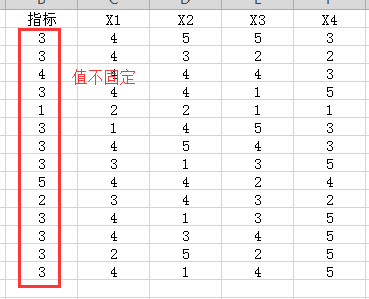pandas如何简单实现excel中的countif函数？np.where(data[data.columns[1:]]> data['指标']).count(1) ---结果都是0

(data[data.columns[1:]]> data['指标']).sum() ---结果也是0

1个回答

``````import pandas as pd

base = [3,3,4,3,1,3,3,3,5,2,3,3,3,3]
x_1 = [4,4,4,4,2,1,4,3,4,3,4,4,2,4]
x_2 = [5,3,4,4,2,4,5,1,4,4,1,3,5,1]
x_3 = [5,2,4,1,1,5,4,3,2,3,3,4,2,4]
x_4 = [3,2,3,5,1,3,3,5,4,2,5,5,5,5]

df = pd.DataFrame({'指标':base, 'X1':x_1, 'X2':x_2, 'X3':x_3, 'X4':x_4})
#print(df)

############### 上面为生成图示数据 #####################

#count_if函数
def countif(line, base, count_column):
line['cnt_if'] = sum(line[count_column] > line[base])
return line

#将count_if应用到每一行
new_df = df.apply(countif, axis=1, args=('指标',['X1', 'X2', 'X3', 'X4'], ))
print(new_df)
``````